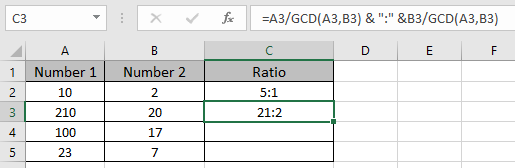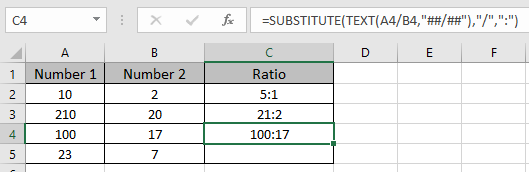# Calculate a ratio of two numbers in Excel

In this article, we will learn how to get a ratio of two numbers in Excel.
In simple words, 10 and 4 are two numbers and we need to get the ratio of the two in the format is 5:2.

Let’s discuss on all the different ways

1. By using GCD function
GCD function returns the greatest common Divisor of the numbers.
Here both number gets divided by the grestest common divisor of the two numbers and represent it in the ratio format using & operator
Use the formula:

= A3/GCD(A3,B3) & ":" & B3/GCD(A3,B3)

A3 : number 1
B3 : number 2
GCD(A3,B3) : returns the greatest common divisor of the two.
& : operator to concatenate the formulaHere is the ratio of the two numbers
2. Using SUBSTITUTE & TEXT function in Excel
Use the formula:

=SUBSTITUTE(TEXT(A4/B4,"##/##"),"/",":")

TEXT(A4/B4,"##/##") : TEXT function represent the operation in the form of ##/##. It basically return the ratio in text format.
SUBSTITUTE function : “/” operator will be replaced by ratio symbol “:”Here is the ratio of the two numbers.

Here is the ratio of the two numbers.
Hope you understood how to get the ratio of the numbers in Excel. Explore more articles here on Excel functions here. Please state your queries or feedback about the article in the comment box below.

Related Articles:

Excel Convert negative number to positive number

How to Use Round function in Excel

Percentage Decrease Change

Popular Articles:

The VLOOKUP Function in Excel

COUNTIF in Excel 2016

How to Use SUMIF Function in Excel

1.